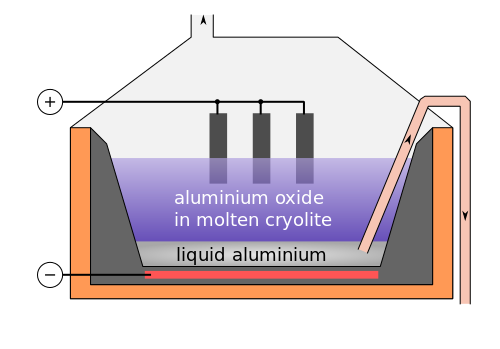Chemistry µGCSE:

Metal Bonding + Extraction

10 quick questions - for GCSE and iGCSE

10 minutes maximum! (can you do it in 5?)

1. Properties of four substances are given in the table below. Which substance is a metallic element?

 Melting point /°C Electrical Conductivity.. ..when solid ..when liquid A -26 low low B 801 low high C 3246 high low D 1436 high high
2. A metallic bond is the …
• A.   mutual attraction of two nuclei for a shared pair of electrons
• B.   transfer of electrons from one atom to another
• C.   attraction of positive ions for a ‘sea’ of delocalized electrons
• D.   attraction of positive ions for negative ions
3. Metals conduct electricity because they contain …..
• A.   delocalized electrons which are free to move
• B.   delocalized electrons which are unable to move
• C.   ions which are free to move
• D.   ions which are in fixed positions
4. Metals are malleable because …
• A.   positive and negative ions are in regular positions and can slide over each other
• B.   positive and negative ions are in regular positions and cannot move
• C.   atoms are arranged in layers so can slide over each other
• D.   atoms are arranged in layers and cannot move
5. The position of metal Q in the reactivity series is shown below:

K    Na    Mg   Fe   (H)   Q    Cu
Which statements about Q and its oxide are correct?

 Reaction of oxide of Q with carbon Reaction of metal Q with dilute acid A no reaction no reaction B no reaction reacts, hydrogen formed C oxide reduced no reaction D oxide reduced reacts, hydrogen formed

6. Three metals are extracted as shown in the table below:

 Metal Method of Extraction R Occurs naturally as the metal S Electrolysis of molten compound T Heat metal oxide with carbon

The order of reactivity of these metals, most reactive first is ….

• A.   R, S, T
• B.   R, T, S
• C.   S, T, R
• D.   T, S, R

7. Iron is extracted from iron(III)oxide in the Blast Furnace by heating with carbon monoxide. The equation for this reaction is:

Fe2O3 + 3CO 2Fe + 3CO2

The substance reduced in this reaction is ….

• A.   iron(III)oxide
• B.   carbon monoxide
• C.   iron
• D.   carbon dioxide
8. Aluminium cannot be extracted from its ore by heating with carbon because aluminium is…..
• A.   less reactive than carbon
• B.   more reactive than carbon
• C.   extracted by electrolysis
• D.   corrosion resistant

9. Aluminium is extracted by the electrolysis of molten aluminium oxide (Al2O3) using the apparatus shown.

Which row below correctly shows the half equations for the reactions occurring at the electrodes?Cepheiden | CC 3.0
 Equation at cathode Equation at anode A 2O2- → O2 + 4e- Al3+ + 3e- → Al B O2- → O + 2e- Al3+ + 3e- → Al C Al3+ + 3e- → Al O2- → O + 2e- D Al3+ + 3e- → Al 2O2- → O2 + 4e-
10. Which of the oxides MgO, CuO and K2O can be reduced by heating with carbon?
• A.   K2O only
• B.   CuO only
• C.   MgO and K2O only
• D.   MgO and CuO only